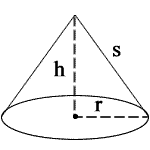## Cone Calculator

 r = h = Units: s = 0 V = 0 L = 0 B = 0 A = 0
» Show Work

r = radius, h = height, s = slant height

V = volume, L = lateral surface area, B = base surface area, A = total surface area

Cones are a pyramids with a circular base.

To calculate the properties of a cone, you must know some of the value of it, like r and s, r and h, r and L, r and V, r and A, h and V,h and s, or s and L follow the formulas below.### ConeFormulas

Cone Volume = (1/3) π r2 h

Cone Surface Area = π r (l + r)

The Area and Volume of a Cone can be calculated in different units by multiplying respective value to the resulting value. These are all the below values are used to convert the units in different units

For example, When r = 3, r = 2,

1. To calculate s when given r and h, use the following formula: √(r2 + h2). When you substitute r and h for their values, you get √(32 + 22), which equals 3.60555 m.
2. To calculate V, use the following formula: (1/3)πr2h. When you substitute r and h for their values, you get (1/3)π322, which equals 18.84956 m3.
3. To calculate L, use the following formula: πrs. When you substitute r and s for their values, you get π * 3 * 3.60555, which equals 33.98151 m2.
4. To calculate B, use the following formula: πr2. When you substitute r for its value, you get π32, which equals 28.27433 m2.
5. To calculate A, use the following formula: L + B. When you substitute L and B for their values, you get 33.98151 + 28.27433, which equals 62.25584 m2.

Thinkcalculator.com provides you helpful and handy calculator resources.